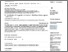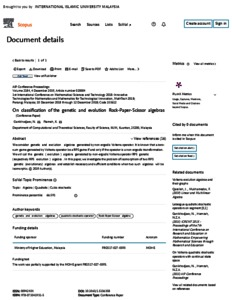# On classification of the genetic and evolution rock-paper-scissor algebras

Ganikhodjaev, Nasir and Ftameh, Kalid (2018) On classification of the genetic and evolution rock-paper-scissor algebras. In: International Conference on Mathematical Sciences and Technology 2018, 10th-12th Dec. 2018, Penang.PDF - Published Version
Restricted to Repository staff onlyPreview
PDF (Scopus) - Supplemental Material

## Abstract

In recent decades in game theory, evolutionary and dynamically aspects have increased in popularity. An evolutionary zero-sum game generated by a Volterra quadratic stochastic operator V is called a Rock-Paper-Scissors (RPS) game if the operator V is a non-ergodic transformation. An algebra with genetic realization (genetic algebra) is an algebra A over the real numbers R which has a finite basis and a multiplication table is generated by the Volterra quadratic stochastic operator. An evolution algebra over the real numbers R is an algebra with a basis on which multiplication is defined by the product of distinct basis terms being zero and the square of each basis element being a linear form in basis elements. We assume that the structure constants in the linear form are defined also by Volterra operator. We consider genetic and evolution algebras generated by non-ergodic Volterra operator. It is known that a zero-sum game generated by Volterra operator be a RPS game if and only if the operator is a non-ergodic transformation. We will call the genetic (evolution) algebra generated by non-ergodic Volterra operator RPS genetic (respectively RPS evolution) algebra. In this paper we investigate the problem of isomorphism of two RPS genetic (evolutionary) algebras and establish necessary and sufficient conditions when two such algebras will be isomorphic.

Item Type: Conference or Workshop Item (Plenary Papers) 4430/67472 Quadratic Stochastic Operator, Rock-Paper-Scissor game, Genetic and Evolution algebras. Q Science > QA Mathematics Kulliyyah of ScienceKulliyyah of Science > Department of Computational and Theoretical Sciences Prof. Nasir Ganikhodjaev 19 Nov 2018 10:09 15 Jan 2020 08:42 http://irep.iium.edu.my/id/eprint/67472View Item